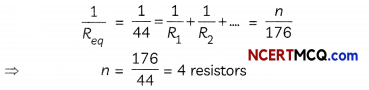## Ohm’s Law

Let us find out if there is any relationship between the potential difference across a conductor And the current through it.

Example 1.
Case-Based:
Set up a circuit as shown in Fig. below, consisting of a nichrome wire XY of length, say 0.5 m, an ammeter, a voltmeter, and four cells of 1.5 V each.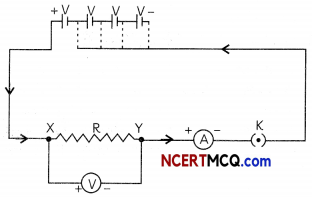First use only one cell as the source in the circuit. Note the reading in the ammeter I, for the current and reading of the voltmeter V for the potential difference across the nichrome wire XY in the circuit. Tabulate them in the Table given.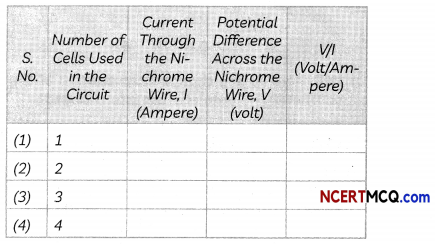Next connect two ceLLs in the circuit and note the respective readings of the ammeter and voltmeter for the values of current through the nichrome wire and potential difference across the nichrome wire. Repeat the above steps using three cells and then four cells in the circuit separately.
(A) Nichrome is an alloy of:
(a) Nickel and Chromium
(b) Nickel, Chromium, and Iron
(c) Nickel, Chromium, Manganese, and Iron
(d) Nickel, Chromium, Magnesium and Iron
(c) Nickel, Chromium, Manganese and Iron

Explanation: Nichrome is an alloy of Nickel, Chromium, Manganese and Iron commonly used in resistances and heating elements.

(B) Select the correct observations when the above activity is repeated with one cell, two cells, three and then four cells:
(I) On increasing the number of cells in the circuit, the reading of the voltmeter increases.
(II) On increasing the number of cells in the circuit, the reading of voltmeter decreas¬es.
(III) On increasing the number of cells in the circuit, the reading of ammeter increases.
(IV) On increasing the number of cells in the circuit, the reading of ammeter decreases.
(a) Both (I) and (III)
(b) Both (I) and (IV)
(c) Both (II) and (III)
(d) Both (II) and (IV)
(a) Both (I) and (III)

Explanation: On increasing the number of cells in the circuit, the reading of voLtmeter increases as the potential difference across the nichrome wire increases. When potential difference across the nichrome wire increases, the current also increases.(C) A student tabulated the voltmeter and am¬meter readings as shown in the table below. What conclusions can be drawn regarding V/I ratio and the nature of the graph?The V/I ratio is a constant for the given nichrome wire. The graph between V and I is a straight line passing through the origin as shown in the graph below:(D) Will there be any change if the position of the voltmeter and ammeter are interchanged?
A voltmeter is a device having high resistance for measuring the potential difference and is always connected in parallel across the component whose potential difference is to be measured. Ammeter is a device having low resistance for measuring the electric current flowing in the circuit. If the ammeter is connected in place of voltmeter, it will get burnt as all the current will flow through it since it has very low resistance. Moreover, connecting a high resistance voltmeter in the circuit, will increase the overall resistance in the circuit and it will not show the correct values.

(E) Assertion (A): The V/I ratio increases linearly with increase in potential difference.
Reason (R): The V-lgraph is a straight line that passes through the origin of the graph.
(a) Both (A) and (R) are true and (R) is the correct explanation of the assertion.
(b) Both (A) and (R) are true, but (R) is not the correct explanation of the assertion.
(c) (A) is true, but (R) is false.
(d) (A) is false, but (R) is true.
(d) (A) is false, but (R) is true.

Explanation: The V-l graph is a straight line that passes through the origin which shows that the V/I ratio for a given nichrome wire is a constant value that does not change. The value of current I increases linearly when the potential difference V is increased.

Ohm’s Law states that temperature and other physical conditions remaining the same, the current passing through a wire is directly proportional to the potential difference across the wire. In other words, V oc I or, V = IR, where V is the potential difference across the wire, I is the current flowing and R is the constant of proportionality, known as the Resistance of the wire.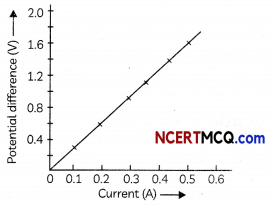## Resistance

It is a property of a wire which retards the flow of the current through the wire. It is due to the opposition encountered by the electrons as the electrons are restrained by the attractive force of the atoms and also due to the collisions with other electrons and with the atoms.

## 1 Ohm

It is the unit of resistance. The resistance of a conductor is said to be 1 Ohm when a potential difference of 1 Volt across the ends of the conductor produces a current of 1 ampere.

## Rheostat

It is a device that is used in an electric circuit to change the resistance in the circuit.

Example 2.
The values of current I flowing in a given resistor for the corresponding values of potential difference V across the resistor are given below: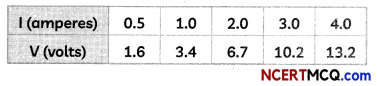Plot a graph between V and I and calculate the resistance of that resistor.
The graph between V and I is drawn below:The resistance of the resistor can be found from the graph by finding the slope of the VI graph.
R = slope of VI graph
= $$\frac{V_{2}-V_{1}}{I_{2}-1_{1}}=\frac{13.2-1.6}{4-0.5}=\frac{11.6}{3.5}$$ = 3.31 OhmExample 3.
How many 176 D resistors (in parallel) are required to carry 5 A on a 220 V line?
Therefore, required resistance R = $$\frac{V}{1}=\frac{220}{5}$$ = 44Ω.